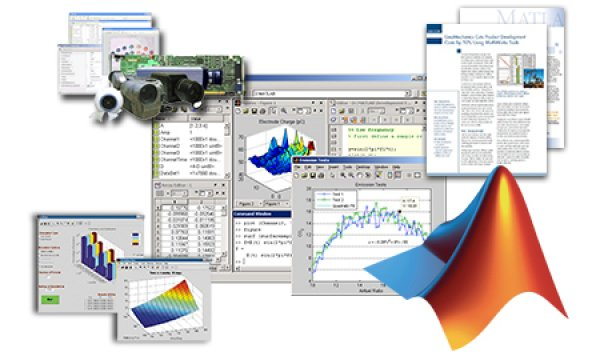FREE MATLAB Interactive Technical Kit

30.03.2012. | Robert Cupec Ispis

MATLAB provides the foundation for making progress in vital areas of engineering and science. Over one million people around the world use MATLAB to help them develop cancer therapies, search for new sources of energy, make our cars safer and more fuel-efficient, and explore outer space. By combining a powerful numeric engine and technical programming environment with interactive exploration and visualization tools, MATLAB has become the language of technical computing.

Download a complimentary interactive MATLAB Technical Kit:

Complete this form today to access the MATLAB Interactive Kit, featuring examples, webinars, technical literature and more.

Recorded Product Presentations

Demonstrations led by MATLAB product experts
• Introduction to MATLAB
• Data Analysis with MATLAB for Excel Users
• Algorithm Development with MATLAB for C/C++ Products
• Introduction to Statistics with MATLAB Products
• Introduction to Optimization with MATLAB Products
• Application Deployment with MATLAB
• MATLAB for Signal Processing
• Image Processing Using MATLAB
• Data Acquisition with MATLAB Products
• Using MATLAB to Develop Financial Products
• Introduction to MATLAB for Life Scientists

User Stories

Concise reports about MathWorks customers solving real-world problems
• Ford Motor Company Develops and Deploys Sound-Quality Metrics with MATLAB
• DEQX Improves Speaker Sound Quality with MATLAB
• Intuitive Analytics Uses MATLAB to Build Quantitative Tools to Help Bond Issuers Manage Risk
• Beth Israel Deaconess Medical Center Improves MRI Accuracy Using MathWorks Tools

Product Data Sheets

Descriptions of featured products in the MATLAB product family
• MATLAB
• Signal Processing Toolbox
• Data Acquisition Toolbox
• Financial Toolbox
• MATLAB Compiler
• Optimization Toolbox⇦ Nazad na pregled obavijesti